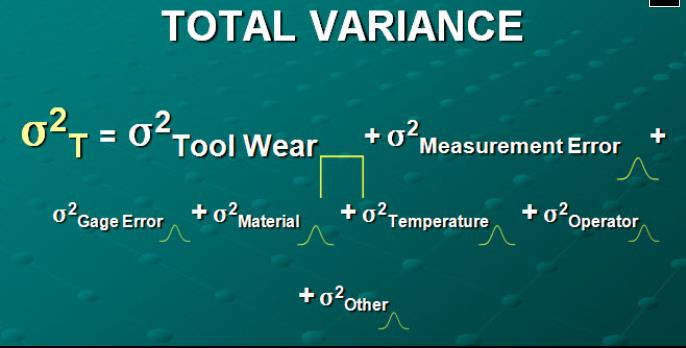# Total Variance Equation

Your process output is not one variance, but a sum of many.

1.When determining process capability, you need to understand the sources of variation. What is the process, and what would one expect the distribution to be? Often the time-ordered run chart has as much to say about the distribution than just jumping to normality checking. Also, can you prepare the total variance equation? What are the expected distributions of each of the contributors of variation? Assuming a process has one net variation is simultaneously assuming that all of the variation in the total variation equation are insignificant except one big factor. Sometimes that is true - sometimes the biggest factor is measurement error, which tends to be normal, and does a great job of masking the true process variation distribution.

So, the first question is: why do you need to know if the distribution is normal? Is it to determine capability? Then you probably jumped ahead a few steps.
-First step is figure out your total variance equation.
-Then, ensure all of the factors that should be held statistically insignificant are - measurement error, gage error, material variation, etc. - so that the resulting variation is the one you are trying to study. If there are more than one factor that are significant, you can get more than one "modes". Is that "not capable" or "not in control"? Of course not - but simpler statistical thinkers may have you thinking that is the case. Fact is, what are the odds that you will only have one significant factor looking at the total variance equation? Pretty slim.
-The next step is to prepare a run chart to see if there was any significant trends, or if the data was truly random and discrete (what you want for a normal distribution).
-Finally, run the data through a distribution fit analysis, and find the best fit. If, as an example, the best fit is p=.6 and the normal curve fit p=.4, you can likely use the normal statistics as an estimate of the curve behavior. But, the best fit is p=.6 and the normal curve fit p=.05, then you need to look at the correct distribution's statistics or...as a last choice, perform transformation.
krichmond0306 likes this.